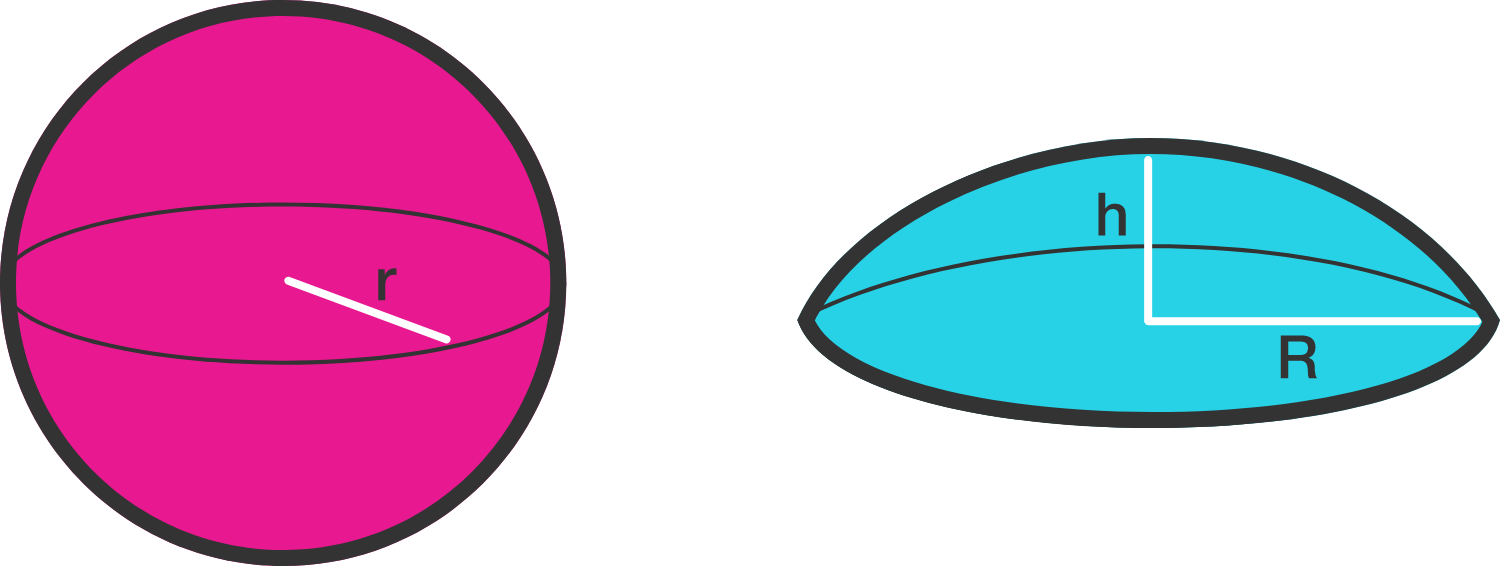# Ball & DomeA sweets shop sells candies in 2 different styles: a spherical ball and a dome. The dome-like shape is a spherical section of a larger sphere with height $h$ and base radius $R,$ as shown above, while the candy ball has radius $r$ with $2r = R + h$.

If both shapes have the same total surface area, what is the ratio $\frac{R}{h}$?

×